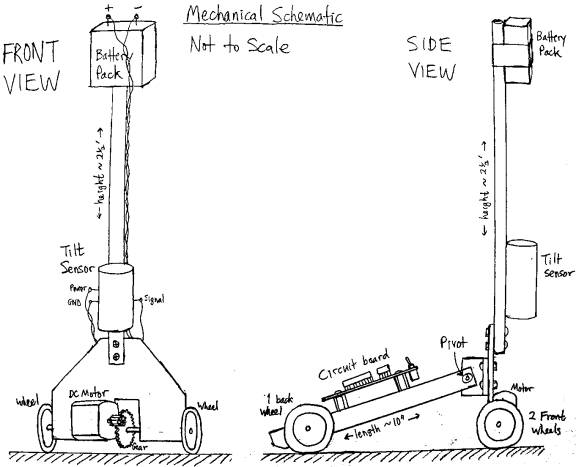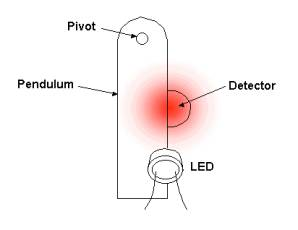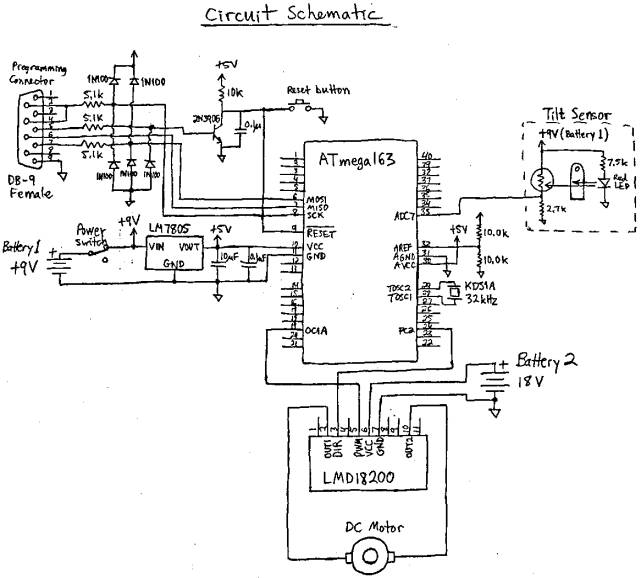EE281 Final Project                           Self-Balancing Robot             Geoffrey Bainbridge

Dec 12, 2001

Introduction

The goal of this project was to build a one-legged robot which balances itself.  The robot has a vertical shaft with two wheels at the bottom.  There is also a “tail” with a third wheel at the end, but this tail is attached through a pivot point, so the third wheel does not help to balance the robot.  The robot can fall forwards or backwards, but not to the side.  It senses its tilt and drives forwards or backwards to compensate.

Feedback control is provided by an ATmega163 microcontroller.  It digitizes the voltage from a tilt sensor on the vertical shaft, and uses this information to control the driving voltage of a DC motor attached to the two front wheels.  The microcontroller is programmable through a serial port, to allow for modifications to the robot or refinements of the control scheme.Mechanics

Tilt is equivalent to sideways gravitational force.  The main difficulty in the control scheme is distinguishing tilt from horizontal acceleration.  If the tilt sensor was mounted at the center of mass of the vertical shaft, then it would be impossible to measure tilt dynamically, because if the robot was tipping over due to gravity, the tilt sensor would be in free fall and register no force at all.  However with the battery pack at the top of the shaft and the tilt sensor near the bottom, the horizontal acceleration due to gravity is reduced, and the sensor is able to detect tilt.  Unfortunately, location at the bottom of the shaft makes the tilt sensor sensitive to horizontal acceleration from the motor.  This unwanted feedback can be mitigated to some extent in processing, but not eliminated, as we shall see later.

The tilt sensor uses an LED and CdS photoresistor to measure the motion of a small strip of metal hanging from a pivot point at the top, as shown below.  When the machine tilts, the strip moves to cover either more or less of the detector, producing an approximately proportional change in resistance. The CdS photoresistor is in series with a larger fixed resistor, so the current through it is approximately constant, and the change in resistance produces an approximately proportional change in the voltage across it.  This voltage is used as the output signal.

Tilt SensorThe metal strip is about ¾” long and has a resonant frequency of 4.8 Hz.  The sensor response peaks sharply at this frequency and then rolls off at 20 dB/decade above.  Attempts to compensate for the sensor response are discussed later, under the heading of Feedback Control.

The motor is a small DC motor weighing about 1 ounce, and is rated for 3-6 V, but will operate over a wider range.  The drive assembly was designed for low friction, and will turn (under no load) with only 0.4 V applied voltage, drawing about 10 mA, for 4 mW power consumption.  The power is supplied by two 9V batteries in series, which can produce a maximum of 500 mA, for a peak power of 9 W.  The small mass and low friction of the motor help to make it respond rapidly and linearly to the driving voltage.  However the total mass of the lower part of the machine is about 5 ounces, which creates a significant inertial load.

The robot was originally intended to be just a vertical shaft with two wheels, but the tail was added to keep it from twisting around the vertical axis.  Any bump or vibration can cause it to lean to one side, which will lift one of the drive wheels off the ground.  With only one wheel touching, it tends to spin around and become uncontrollable.  The tail prevents this from happening.

Electronics

The circuit is built around an ATmega163 microcontroller.  128 times per second it samples the voltage from the tilt sensor and generates an appropriate value for the motor torque to correct the tilt.  The motor drive signal comes out on two pins.  OC1A is a pulse-width-modulated signal which controls the magnitude of the drive voltage.  PC2 is binary hi/lo signal which indicates the direction.  These control signals go to an LMD18200 motor driver chip, which switches power from a separate 18V supply into the motor.The system uses 3 batteries and 3 different supply voltages.  Two batteries provide unregulated 18V for the motor, and the third battery provides regulated 5V for the microcontroller and unregulated 9V for the tilt sensor.  9V is used for the tilt sensor, since a higher supply voltage allows us to put a larger fixed resistor in series with the photoresistor, and get a wider range of linear voltage output from the tilt sensor than would be allowed by a 5V supply.  The 9V supply is not regulated, since the sensor operates over a wide voltage range and is automatically calibrated by the microcontroller (see description of integral center adjustment under Feedback Control).  The motor supply does not need to be regulated either, but it needs to be separate, so that the large switching currents do not feed back to the tilt sensor input.

The circuit includes a serial port programming interface to allow for software changes.

Software

The control program was written in C (rather than assembly language) because the program requires math functions not directly supported in AVR assembler (such as division), and speed is not much of an issue.  Since we sample 128 times per second, and the processor runs at 1MHz, there are about 8k cycles available to compute the output before the next input comes in.

The main program initializes the system and then goes into an endless wait loop.  After that everything happens on the Timer2 overflow interrupt.  Timer2 runs off an external 32k crystal, since the timing of the microcontroller’s internal LC oscillator is not very reliable.  Its nominal clock rate is 1 MHz, but I measured it actually running at 790 kHz.  Good timing is required for the matched filter part of the signal processing (see next section).  If the sample rate is off, the filter will not be matched to the system response.

Other than that, the software architecture is straightforward, just a linear sequence of commands.  For details see the code listing (with comments) at the end of this report.

Feedback Control

The system uses proportional-integral-derivative (PID) control.  The most basic part is the proportional response.  When the sensor measures a tilt, a proportional voltage is applied to the motor, in the appropriate direction so as to correct the tilt and balance the machine.  The larger the constant of proportionality, the stronger the correction will be.  However this does not necessarily mean that the system will be more stable, since there is a phase shift between the sensor measurement and the response of the mechanical system.

With a simple, uncompensated proportional response, the system oscillates at the sensor resonance frequency of 4.8 Hz.  This oscillation arises spontaneously and grows to the point where it paralyzes the system.  A signal processing correction must be applied to fix this.

The first thing I tried was a matched filter.  The tilt sensor is essentially a pendulum, so it has a decaying sinusoidal response, with period T.  We can take advantage of the symmetry of the impulse response to cancel out the ringing.  In the resonant tail of the response, V(t) = -aV(t-T/2) , where a is a decay constant for the oscillation.  Therefore the ringing can be cancelled by convolution with a two-tap FIR filter whose values are f(0) = 1/(a+1) and f(T/2) = a/(a+1).  I measured the filter impulse response by tapping the tilt sensor and recording the output on a digital scope.  This gave values of T = 0.209 s, and a = 0.78.

The matched filter was effective in controlling the sensor oscillation.  However the system then started to oscillate at a lower frequency, around 1 Hz.  This was caused by overshoot of the motor.  As the bottom of the machine caught up with the top and passed the center point, it had a lot of momentum, and there was no force to stop it (since the tilt was zero).  The motor would overshoot, and a restoring force would be applied only after some delay, due to the matched filter.  (Any convolution filter introduces delay.)  As a result, the overshoot would go farther each time, and the oscillation would grow.

The only cure for this was to use derivative damping.  A term was added to the motor output proportional to the first derivative of the tilt measurement, which acted to slow the motor down as the machine reached the balance point.  This eliminated the low-frequency oscillation.  However it meant the FIR filter was no longer matched to the system response.  An unmatched filter is worse than no filter at all, so I had to stop using it.  Fortunately, the derivative term also damped out system resonances at higher frequencies, so there was no need for the filter.  In particular, it suppressed the tilt sensor resonance, not by damping the sensor, but by preventing the motor from jerking suddenly and exciting this resonance in the first place.

The final element of the control system is integral center adjustment.  This is a way of automatically calibrating the center balance point.  If the designated center value of the tilt center does not correspond the to actual balance point, then gravity will tend to pull the machine more to one side.  Therefore if the integral of the measured tilt is non-zero, this indicates the center value needs adjustment, and the sign of the integral indicates which direction to move the center.  Somewhat counterintuitively, we must move the center value so as to increase the average tilt.  This will make the machine pull harder towards the balance point and correct itself.

The integral adjustment should ideally make the machine find the true, gravity-referenced balance point.  However in reality a fudge factor (called BAL_ADJ) must be added to make it work out.  I attribute this to non-linearity of the tilt sensor.  Even if the CdS cell resistance is exactly proportional the tilt angle, the voltage drop across it will not be, since it equals Vcc*R/(R+R0), where Vcc is the supply voltage, R is the CdS cell resistance, and R0 is a fixed resistance in series with it.  This means the tilt sensor has higher gain in one direction than the other, and so the integral will be skewed to one side.

System Performance

The loop gain is set just below the threshold of spontaneous oscillation at the sensor frequency.  The machine almost balances itself, in that when it starts to tilt, the bottom moves to catch up with the top.  However it never quite makes it, and the machine slowly falls over while moving sideways.  If the top of the shaft is held, and moved back and forth at moderate speeds (a slow walking pace), the bottom is able to keep up with it, and track the movements of the top with some overshoot, but no oscillation.

At first I thought that if I could just control resonances better, and crank up the gain without oscillation, then the robot would balance itself.  Now I realize the problem is more fundamental, namely Einstein’s principle which states that there is no way to distinguish between gravity and acceleration without an external reference frame.  Gravity causes the top of the robot to accelerate forward or back.  The sensor measures the tilt, and the bottom tries to catch up with the top, but it never can, because in order to do that it would have to accelerate faster than the gravitational tilting force, and this would cause the tilt sensor to swing back the other way before the machine had actually balanced itself.

I can think of two solutions to this.  One is to use an external tilt reference, such as an optical system that tracks a fixed reference point.  The other, more elegant solution is to put a second, identical tilt sensor on the tail of the machine, which does not tilt, but has the same horizontal acceleration.  Then the fixed sensor voltage could be subtracted from the tilting sensor voltage (after low-pass filtering?) to give a tilt measurement independent of acceleration.

Program Code

//  BALANCER

//  Geoff Bainbridge

//  Dec. 11, 2001

//  PID control code for self-balancing one-legged robot

//  for ATmega163 microcontroller

//  Final project for EE281

#include <io.h>

#include <sig-avr.h>

#include <interrupt.h>

// DEFINE CONSTANTS AND VARIABLES

#define BAL_ADJ -5                  // center calibration factor

volatile unsigned char temp;

volatile unsigned char center;      // tilt sensor value at center balance point

volatile int offset;                // offset = tilt - center

volatile unsigned char pwmlevel;    // magnitude of motor drive output

volatile unsigned char direction;   // direction of motor drive output

volatile unsigned char *data;       // ring buffer stores tilt values

volatile unsigned char i;           // index to ring buffer

volatile unsigned char j;           // index to convolution filter

volatile unsigned int sum;          // sum of past tilt values for block average

volatile unsigned char tilt;        // current tilt (filtered)

volatile unsigned char tilt0;       // previous tilt (filtered)

volatile char dtilt;                // 1st derivative of tilt

volatile int torque;                // torque applied to motor (signed value)

// MAIN PROGRAM

// initializes the system, then enters an endless wait loop;

// everything happens on interrupts.

int main(void)

{

outp(0xFF, DDRC);                // set port C as output (motor direction)

outp(0xFF, DDRD);                // set port D as output (PWM)

outp(231, ADMUX);                // ADC settings: 8-bit, 2.56V internal ref,

// read pin A7

outp(224, ADCSR);                // Enable ADC, start conversion, free run

outp(1|(1<<7), TCCR1A);          // set timer1 as 8-bit PWM

outp(0, OCR1AL);                 // set default pulse width = zero

outp(1, TCCR1B);                 // set timer1 to increment at internal clock

// rate.  PWM pulse rate = 1 MHz / 512 =

// about 2 kHz.

outp((1<<3), ASSR);              // run timer2 from external 32kHz oscillator

outp(1, TCCR2);                  // run at 32kHz, so timer2 overflows 128

// times per second.

outp((1<<6), TIMSK);             // enable timer2 overflow interrupt

temp = inp(TCNT2);               // delay to allow ADC startup

while( inp(TCNT2)==temp ) {}

while( inp(TCNT2)!=temp ) {}

center = inp(ADCH);              // set center = current tilt sensor value

offset = 0;                      // initialize variables

pwmlevel = 0;

direction = 0;

data = (unsigned char *) malloc(256);   // get memory for ring buffer

i=0;                             // initialize index to ring buffer

sei();                           // enable interrupts

while(1) {}                      // wait for interrupt (every 1/128 second)

return 0;

}

// PID FEEDBACK CONTROL

// 128 times per second, read tilt sensor and set motor drive output.

SIGNAL(SIG_OVERFLOW2)

{

tilt0 = tilt;                    // save last tilt value

i++;                             // increment ring buffer index

data[i] = inp(ADCH);             // read tilt sensor value from ADC

// FILTERING: Try to suppress resonances to avoid oscillation.

// I tried different filters; three versions are shown here.

// The filters were matched to the system response without

// derivative damping.  However when I added the derivative

// term, then filters were no longer matched, so they did

// more harm than good, and I had to stop using them.

// Fortunately derivative damping controls the resonances

// pretty well by itself , so I didn't need a filter in the

// final version.

// Version 1: no filter

tilt = data[i];

// Version 2: 2-tap FIR inverse filter

//   temp = i-13;

//   tilt = 0.56*data[i] + 0.44*data[temp];

// Version 3: inverse + block average

//   sum = 0;

//   for (j=0; j<6; j++)

//   {

//      temp = i-j;

//      sum += 7*data[temp];

//   }

//   for (j=13; j<19; j++)

//   {

//      temp = i-j;

//      sum += 5*data[temp];

//   }

//   tilt = sum/72;

// TORQUE is function of tilt and derivative (tilt rate).

// Multiplier constants control loop gain.  They are set just under

// the threshold of spontaneous oscillation.

//

// BAL_ADJ is an adjustment which seems to be necessary to keep the

// balance point from progressively drifting to one side.  I think

// this happens because the tilt sensor has slightly higher gain on

// one side than the other, and this skews the integral center

dtilt = tilt - tilt0;      // derivative = current tilt - last tilt

torque = 3*(tilt - center)/2 + BAL_ADJ + 4*dtilt;

if (torque > 0)            // find direction and magnitude of torque

{

pwmlevel = torque;

direction = 0;

}

else

{

pwmlevel = -torque;

direction = (1<<2);

}

outp(pwmlevel, OCR1AL);    // output to motor driver

outp(direction, PORTC);

// CENTER ADJUSTMENT: This is the integral part of the PID control.

//

// If the machine is consistently tilted more to one side,

// then move the center towards the other side.  This will

// make the machine pull harder towards center and balance

// itself.

if (data[i] > center)      // Offset variable stores tilt history.

offset++;               // Here we don't care how far it's tilted,

else if (data[i] < center) // just which way.  We don't use proportional

offset--;               // correction, because if we did, the

// correction would go slower and slower as

// it approached the balance point, and never

// actually reach it.

if (offset > 26)           // If the system has spent 26 more sample periods

{                          // (1/5 sec) more on one side than the other,

center --;              // then adjust the center.  26 samples equals one

offset = 0;             // full period of oscillation of the tilt sensor.

}                          // If we used a shorter time, then we would be

if (offset < -26)          // adjusting the center on every swing of the tilt

{                          // sensor.

center ++;

offset = 0;

}

}